SLPS206B August   2009  – November 2016

PRODUCTION DATA.

1. 1Features
2. 2Applications
3. 3Description
4. 4Revision History
5. 5Specifications
6. 6Device and Documentation Support
7. 7Mechanical, Packaging, and Orderable Information

#### パッケージ・オプション

デバイスごとのパッケージ図は、PDF版データシートをご参照ください。

• DQG|8

## 5 Specifications

### 5.1 Electrical Characteristics

TA = 25°C (unless otherwise stated)
PARAMETER TEST CONDITIONS MIN TYP MAX UNIT
STATIC CHARACTERISTICS
BVDSS Drain-to-source voltage VGS = 0 V, ID = 250 μA 25 V
IDSS Drain-to-source leakage current VGS = 0 V, VDS = 20 V 1 μA
IGSS Gate-to-source leakage current VDS = 0 V, VGS = +16 / –12 V 100 nA
VGS(th) Gate-to-source threshold voltage VDS = VGS, ID = 250 μA 1.7 2 2.3 V
RDS(on) Drain-to-source on resistance VGS = 4.5 V, ID = 10 A 12 15
VGS = 10 V, ID = 10 A 8 10
gfs Transconductance VDS = 15 V, ID = 10 A 30 S
DYNAMIC CHARACTERISTICS
CISS Input capacitance VGS = 0 V, VDS = 12.5 V, f = 1 MHz 440 570 pF
COSS Output capacitance 330 430 pF
CRSS Reverse transfer capacitance 33 43 pF
Rg Series gate resistance 0.8 1.6 Ω
Qg Gate charge total (4.5 V) VDS = 12.5 V, ID = 10 A 2.9 3.8 nC
Qgd Gate charge gate-to-drain 0.7 nC
Qgs Gate charge gate-to-source 1.5 nC
Qg(th) Gate charge at Vth 0.9 nC
QOSS Output charge VDS = 12.5 V, VGS = 0 V 6.5 nC
td(on) Turnon delay time VDS = 12.5 V, VGS = 4.5 V, ID = 10 A
RG = 2 Ω
5.3 ns
tr Rise time 7.8 ns
td(off) Turnoff delay time 6 ns
tf Fall time 3.1 ns
DIODE CHARACTERISTICS
VSD Diode forward voltage IS = 10 A, VGS = 0 V 0.85 1 V
Qrr Reverse recovery charge VDD = 12.5 V, IF = 10 A, di/dt = 300 A/μs 11.7 nC
trr Reverse recovery time VDD = 12.5 V, IF = 10 A, di/dt = 300 A/μs 15.5 ns

### 5.2 Thermal Information

TA = 25°C (unless otherwise stated)
PARAMETER MIN TYP MAX UNIT
R θJC Junction-to-case thermal resistance(1) 3.5 °C/W
R θJA Junction-to-ambient thermal resistance(1) (2) 55 °C/W
RθJC is determined with the device mounted on a 1-in2 2-oz Cu pad on a 1.5-in × 1.5-in 0.06-in thick FR4 board. RθJC is specified by design while RθJA is determined by the user’s board design.
Device mounted on FR4 Material with 1-in2 of 2-oz Cu.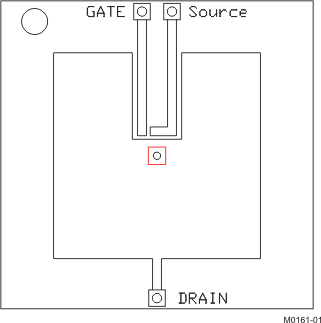Max RθJA = 55°C/W when mounted on 1-in2 of 2-oz Cu.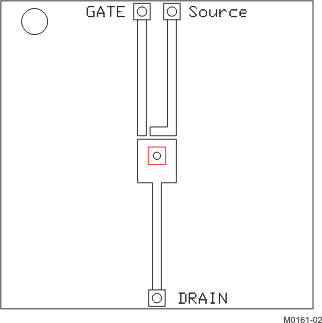Max RθJA = 160°C/W when mounted on minimum pad area of 2-oz Cu.

### 5.3 Typical MOSFET Characteristics

TA = 25°C (unless otherwise stated)Figure 1. Transient Thermal Impedance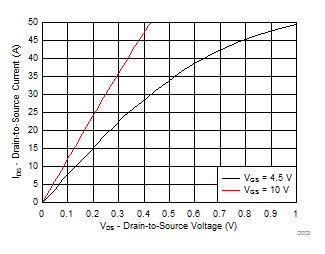Figure 2. Saturation Characteristics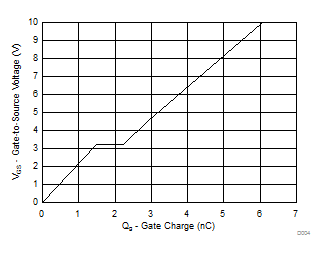VDS = 12.5 V, ID = 10 A
Figure 4. Gate Charge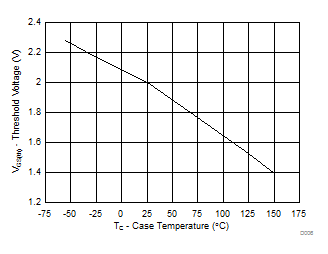ID = 250 µA
Figure 6. Threshold Voltage vs Temperature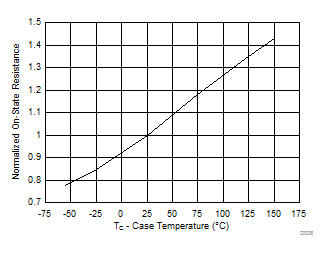ID = 10 A, VGS = 10 V
Figure 8. On Resistance vs Temperature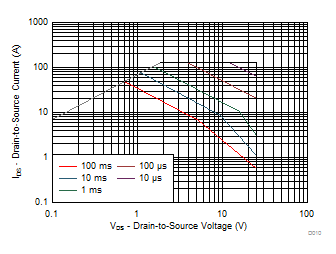Single pulse, max RθJC = 3.5°C/W
Figure 10. Maximum Safe Operating Area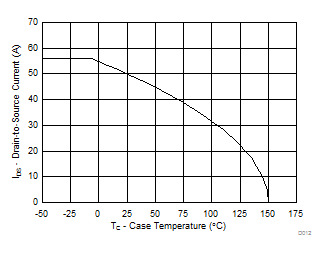Figure 12. Maximum Drain Current vs Temperature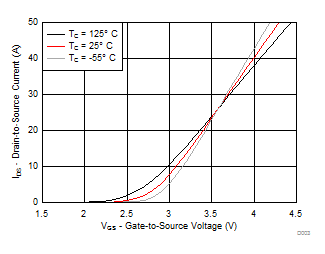VDS = 5 V
Figure 3. Transfer Characteristics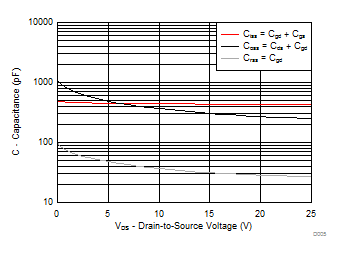Figure 5. Capacitance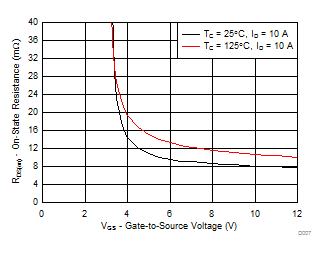Figure 7. On Resistance vs Gate Voltage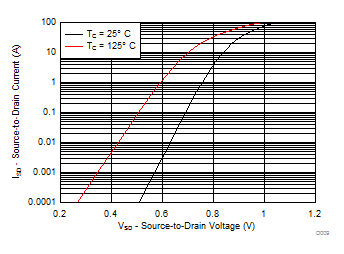Figure 9. Typical Diode Forward Voltage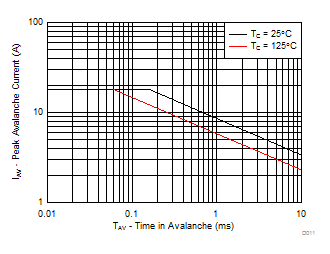Figure 11. Single Pulse Unclamped Inductive Switching